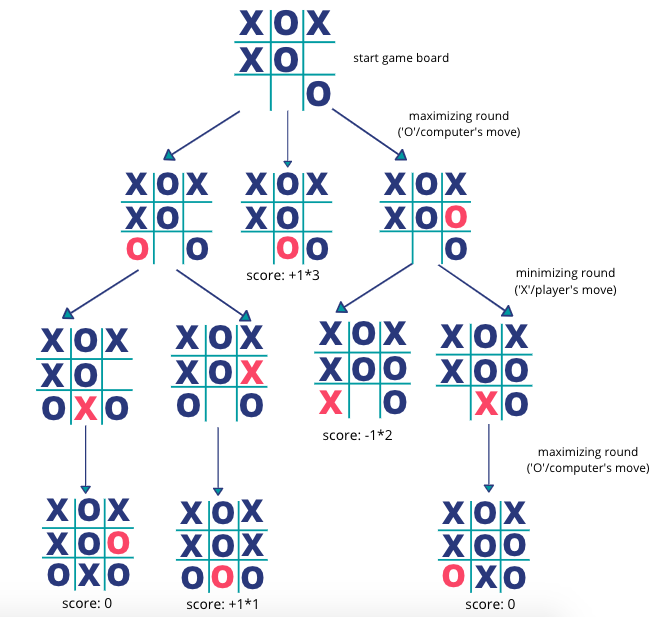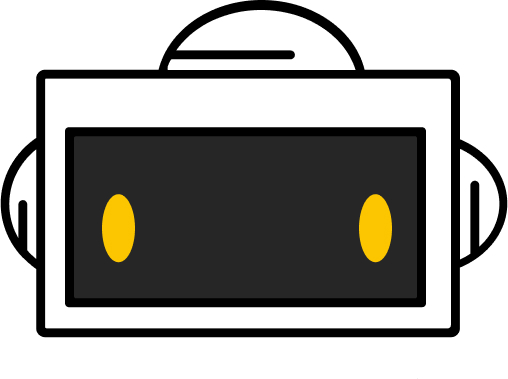# 5. (Optional) AI Move

Workshop Resources

### Let’s make the computer think

Previously, we used a Random object to randomly generate a move for the computer. As a result, the competitiveness of the computer is fairly low.

In this exercise, we want to increase the difficulty of the game by making optimal decisions for the computer.

We will do so by adding artificial intelligence into our program with the use of the Minimax algorithm (a well-defined procedure that allows computers to solve problems).

### Minimax Algorithm

Minimax is an algorithm that is used in two-player games to make optimal decisions for a player.

• The two players are labeled as maximizer and minimizer respectedly. While the maximizer maximizes its chance to win, the minimizer tries to minimize its loss.
• The algorithm examines all the possible future game states based on the current game board assuming both the maximizer and minimizer will pick the move that benefits them the most.
• In our case, we pick the computer to be the maximizer, and the player to be the minimizer. We will attempt to make optimal decision for the computer by maximize its chance to win!

### How will Minimax Algorithm work in TicTacToe?

• We examine all the possible moves of "X" and "O" and give a score to a game board if there is a winner or tie.

• Since we want the computer to win with the fewest steps possible, we design the score for the game boards as the following:

• If computer wins, calculate the score with the formula 1 * (number of available spots on the board + 1).

• If the player wins, calculate the score with the formula -1 * (number of available spots on the board + 1).

• If there is a tie, the score is 0.

• Note that by giving larger scores to game states where the computer can win with fewer steps, we are teaching our code to pick the optimal move for the computer.

Let’s look at an example below:1. In the first row, we considered the 3 possible moves for the computer "O", who is the maximizer.

2. We examine all the game states until all the moves end will a computer win, player win, or a tie. We then give them their corresponding score.

For example, in the second board in row 1, the computer wins by placing "O" at position 8. That state will then be given a score of 1 * (number of available spots on the board + 1) = 1 * (2+1) = 3.

3. At game states that don’t have a winner or a tie, we pick the smallest score during minimizing rounds (when "X" make a move), and the largest score during maximizing rounds (when "O" make a move).

4. If you follow along through the maximizing/minimizing rounds on the picture above, you should note that the optimal move for the computer is to place "O" at position 8, allowing the computer to win with 1 move from the starting game board.

### Code Structure

In activity-3, you wrote the method int getComputerMove(String[] curBoard) to randomly generate a spot for the computer. Let’s write another method called getComputerMoveAI(String[] curBoard) that returns the optimal move for the computer by calling the int minimax(String[] curBoard, boolean isMaximizing) method.

int getComputerMove(String[] curBoard){
// 1. this method calls minimax() on all the possible moves the computer can pick
// 2. it takes the maximum out of all of them
// 3. return the optimal move
}
int minimax(String[] curBoard, boolean isMaximizing){
// 1. In the maximizing round, it calls minimax() on all the possible moves for the computer, "O", return the maximum score
// 2. In the minimizing round, it calls minimax() on all the possible moves for the player, "X", return the minimum score
}


### Write the Method getComputerMoveAI()

1. For each available spot on the game board, place "O" at that spot and get the score for that board by calling minimax().
1. Track the largest score and its corresponding position of the board at each iteration. Return the position with the largest score.

### Write the Method minimax()

As we discussed above, the minimax() method has the header int minimax(String[] curBoard, boolean isMaximizing).

1. Call getWinner() on the board to check if there is a winner. If so, return the corresponding score.

(Score: computer wins (1 * number of available spots on the board + 1), player wins (-1 * number of available spots on the board + 1), tie (0)).

2. Get Minimax Score

• If it’s maximizer’s turn ("O"), for each available spot on the game board, place "O" at that spot and get the score for that board by calling minimax().

• If it’s minimizer’s turn ("X"), for each available spot on the game board, place "X" at that spot and get the score for that board by calling minimax().

1. Return Largest Score
• If it’s maximizer’s turn ("O"), track the largest score and the corresponding position at each iteration, and return that score.
• If it’s minimizer’s turn ("X"), track the smallest score and the corresponding position at each iteration, and return that score.

Copy and paste your two methods below main().

Click Run to test around your methods. We provided a given test of the example of the picture above.

You can plug in different boards to test if you get a desirable output.

### Update the Program

After testing the methods, update all the method calls of getComputerMove() to getComputerMoveAI() in your TicTacToe program.

It should be way more challenging to win this time around😀!You did it!
Workshop completed!